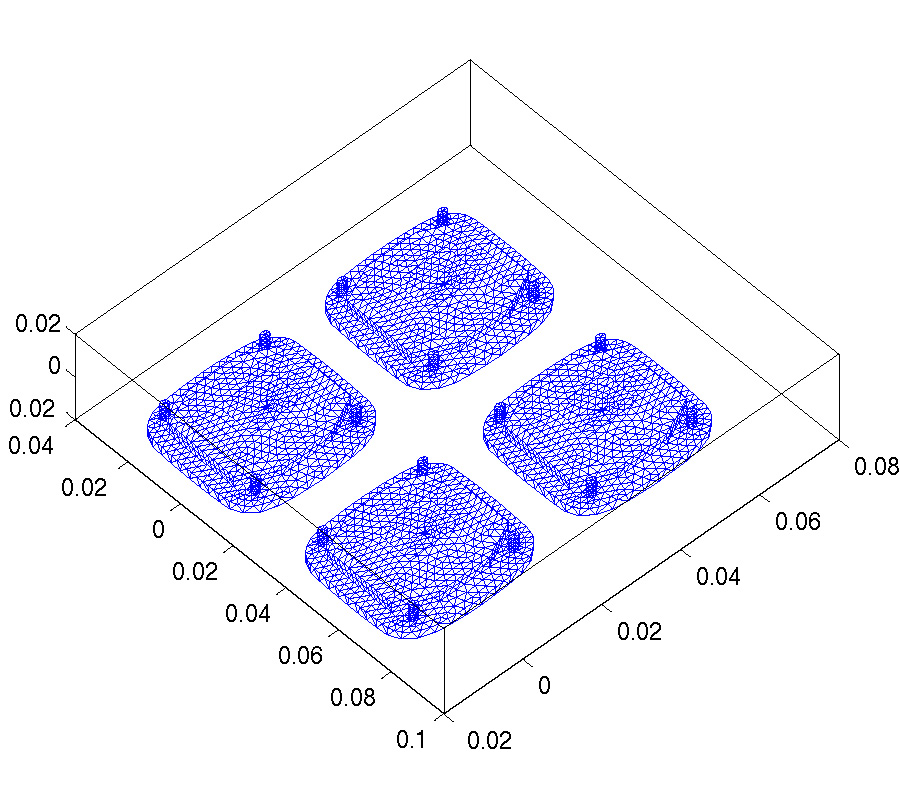# Vol. 71

Front:[PDF file] Back:[PDF file]
Latest Volume
All Volumes
All Issues
2018-07-30

#### A T-Matrix Solver for Fast Modeling of Scattering from Multiple PEC Objects

By Lin E. Sun
Progress In Electromagnetics Research M, Vol. 71, 85-94, 2018
doi:10.2528/PIERM18040606

## Abstract

T matrix characterizes the scattering property of a single PEC object and does not depend on the incidence. In this work, we propose a method to derive a reduced-order T matrix for a single 3D PEC object with arbitrary shape. The method is based on the vector addition theorem and the conventional EFIE, MFIE or CFIE methods. Given the T matrix for a PEC object, the scattered fields can be directly calculated from any incidence. For multiple objects, a matrix equation system is built based on the T-matrix and the position of each object. Finally, numerical examples show the accuracy and efficiency for solving the scattering of both spherical and non-spherical arrays. Compared to the moment methods, the computational cost of solving the final matrix equation is reduced by several orders of magnitude.

## Citation

Lin E. Sun, "A T-Matrix Solver for Fast Modeling of Scattering from Multiple PEC Objects," Progress In Electromagnetics Research M, Vol. 71, 85-94, 2018.
doi:10.2528/PIERM18040606
http://www.jpier.org/PIERM/pier.php?paper=18040606

## References

1. Waterman, P. C., "Matrix formulation of electromagnetic scattering," Proc. IEEE, Vol. 53, 805-812, 1965.
doi:10.1109/PROC.1965.4058

2. Peterson, B. and S. Strom, "T matrix for electromagnetic scattering from an arbitrary number of scatterers and representations of E(3)*," Physical Review, Vol. 8, No. 10, 3661-3678, Nov. 1973.

3. Wang, Y. M. and W. C. Chew, "An efficient algorithm for solution of a scattering problem," Microwave and Optical Technology Letters, Vol. 3, No. 3, 102-106, Mar. 1990.
doi:10.1002/mop.4650030309

4. Wang, Y. M. and W. C. Chew, "A recursive T-matrix approach for solution of electromagnetic scattering by many spheres," IEEE Trans. on Antennas and Propagation, Vol. 41, No. 12, 1633-1639, Dec. 1993.
doi:10.1109/8.273306

5. Gurel, L. and W. C. Chew, "A recursive T-matrix algorithm for strips and patches," Radio Science, Vol. 27, No. 3, 387-401, May-Jun. 1992.
doi:10.1029/91RS03054

6. Chew, W. C., "Vector addition theorem and its diagonalization," Commun. Comput. Phys., Vol. 3, No. 2, 330-341, Feb. 2008.

7. Chew, W. C., Waves and Fields in Inhomogeneous Media, IEEE Press, 1995.

8. Sun, L. E. and W. C. Chew, "An efficient T-matrix method for analyzing the electromagnetic scattering of multiple PEC objects," Proc. of URSI General Assembly, San Diego, CA, Jul. 2008.

9. Mackowski, D. W. and M. I. Mishchenko, "Calculation of the T-matrix and the scattering matrix for ensembles of spheres," J. Opt. Soc. Am. A, Vol. 13, 2266-2278, 1996.
doi:10.1364/JOSAA.13.002266

10. Xu, H. X., "Calculation of the near field of aggregates of arbitrary spheres," J. Opt. Soc. Am. A, Vol. 21, No. 5, 804-809, May 2004.
doi:10.1364/JOSAA.21.000804

11. Forestiere, C., G. Iadarola, L. D. Negro, and G. Miano, "Near-field calculation based on the T-matrix method with discrete sources," Journal of Quantitative Spectroscopy & Radiative Transfer, Vol. 112, 2384-2394, 2011.
doi:10.1016/j.jqsrt.2011.05.009

12. Zhang, Y. J. and E. P. Li, "Fast multipole accelerated scattering matrix method for multiple scattering of a large number of cylinders," Progress In Electromagnetics Research, Vol. 72, 105-126, 2007.
doi:10.2528/PIER07030503

13. Hao, S., P. G. Martinsson, and P. Young, "An efficient and highly accurate solver for multi-body acoustic scattering problems involving rotationally symmetric scatterers," Computers & Mathematics with Applications, Vol. 69, 304-318, 2015.
doi:10.1016/j.camwa.2014.11.014

14. Kim, K. T. and B. A. Kramer, "Direct determination of the T-matrix from a MOM impedance matrix computed using the Rao-Wilton-Glisson basis function," IEEE Trans. on Antennas and Propagation, Vol. 61, No. 10, 5324-5327, 2013.
doi:10.1109/TAP.2013.2273485

15. Gimbutas, Z. and L. Greengard, "Fast multi-particle scattering: A hybrid solver for the Maxwell equations in microstructured materials," Journal of Computational Physics, Vol. 232, 22-32, 2013.
doi:10.1016/j.jcp.2012.01.041